3.2.2 Ideal Gas Equation

Ideal Gas Equation

For a gas at constant temperature and pressure, the number of moles n is directly proportional to the volume V of the gas

• This can be expressed in equation form as: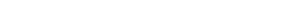• This means that two different gases of equal temperatures, pressures and volumes have the same number of particles N
• Note that the number of particles N is directly proportional to the number of moles n

Equation of State of an Ideal Gase

• Boyle's Law, Charles's Law and Gay-Lussac's law can be combined with Avogadro’s law to give a single constant, known as the ideal gas constant, R
• Combining the four equations leads to the equation of state of an ideal gas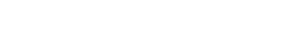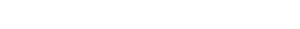• Where:
• p = pressure in pascals (Pa)
• V = volume in metres cubed (m3)
• T = temperature in kelvin (K)
• n = number of moles in the gas (mol)
• R = 8.31 J K–1 mol–1 (ideal gas constant)

Worked Example

A gas has a temperature of –55°C and a pressure of 0.5 MPa. It occupies a volume of 0.02 m3.

Calculate the number of gas particles.

Step 1: Write down the known quantities

• Temperature, T = –55°C = 218 K
• Pressure, p = 0.5 MPa = 0.5 × 106 Pa
• Volume, V = 0.02 m3

Note the conversions:

• The pressure p must be converted from megapascals (MPa) into pascals (Pa)
• The temperature must be converted from degrees Celsius (°C) into kelvin (K)

Step 2: Write down the equation of state of ideal gasesStep 3: Rearrange the above equation to calculate the number of moles n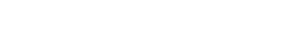Step 4: Substitute numbers into the equation

• From the data booklet, R = 8.31 J K–1 mol–1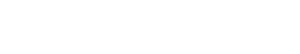n = 5.5 mol

Step 5: Calculate the number of particles N

• Write down the relationship between number of particles and number of moles

N = nNA

• From the data booklet, NA = 6.02 × 1023 mol–1 (Avogadro constant)

N = 5.5 mol × (6.02 × 1023) mol–1

N = 3.3 × 1024

Exam Tip

When using the equation of state of ideal gases, always remember to convert temperatures from degrees Celsius (°C) to kelvin (K).

Note that the number of moles n is not the same as the number of particles N:

• When a question asks to calculate the number of particles in a sample of gas, you should first use the equation of state to determine the number of moles n of the gas, and then calculate the number of particles using N = nNA
• If a question gives the number of particles in a sample of gas instead of the number of moles, you should first use n = N/NA to calculate the number of moles of the gas, and then use the equation of state to perform any further calculation (e.g. volume, pressure, etc.)
CloseClose